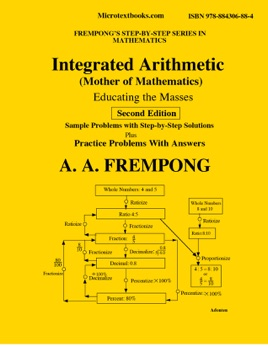• \$8.99

## Publisher Description

Integrated Arithmetic covers: Basic Definitions ;Terminology; and Types of Numbers; Writing Whole Numbers Using Numerals and Words; Basic Operations and Properties; Order of Operations and Evaluation of Arithmetic Expressions; Rounding-off Whole Numbers and Decimals; Estimation; Prime Numbers, Divisibility Rules; Prime Factorization; Least Common Multiple (LCM); Operations on Fractions and Mixed Numbers; Addition and Subtraction of Fractions; Comparison of Fractions and Subtraction of Mixed Numbers; Multiplication and Division: of Fractions and Mixed Numbers; Operations on Decimals; Comparison of Decimals ; Complex Decimals; Dividing Decimals; Converting Fractions to Decimals; Ratio and Proportion; Proportion Problems; Percent (%) and Calculations Involving Percent; Averages; Profit and Loss ; Areas and Perimeters; Bar, Line and Circle (Pie) Graphs; Scientific Notation; Measurements. Every home must have a copy of this book on the living room table